Next: Getting started: Hardware Up: Getting started: Programming Previous: Example: Using approximate

## Morphological processes and reaction-diffusion systems

Turing suggested that the mechanisms of morphogenesis can be explained by ``reaction-diffusion'' equations (see ). These are systems of partial differential equations of the formwhere the vector-valuedat each time t describes the changing spatial concentrations of a set of chemical substances called ``morphogens''. The resulting Turing patterns for various reaction specifications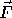have been widely simulated and have been shown to produce regular patterns reminiscent of zebra stripes and leopard spots. Whether or not reaction-diffusion mechanisms are the actual phenomena in such biological systems,simulation of such systems does tend to generate regular patterns that appear as standing-wave solutions to the equations.

Interestingly, the resulting Turing patterns are often independent of the initial state of the system. Starting from a random configuration and evolving the equation eventually results in regular patterns in the concentrations of the morphogens. Figure 2 shows one such set of patterns, obtained from a random initial distribution in a system that evolves according to a system of reaction-diffusion equations called the Gray-Scott model. We have built a simulation environment for exploring these kinds of reaction models. Figure 2 shows a sample result.Figure 2: Simulation of the Gray-Scott model starting from a random initial configuration (left) and evolving to regular spots after 20,000 time steps (right). There are 2500 particles randomly packed in a square of size 1, starting with values of theandmorphogens randomly assigned between 0 and 1. Each particle's neighbors are those within a distance of 0.05 from it. The constants for the Gray-Scott model here are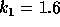,,,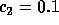, and integration is performed using the forward-Euler method with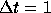. The different colors of the particles indicate different values of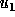.

This suggests the intriguing possibility of organizing amorphous computing systems by starting with the particles in a random state and solving a discrete analog of a reaction-diffusion system. The resulting regularly spaced ``blobs'' of particles could then serve as organizing centers for subsequent stages of computing.Next: Getting started: Hardware Up: Getting started: Programming Previous: Example: Using approximate

Gerald Jay Sussman
Thu Jun 27 16:56:19 EDT 1996